# Electronics and Communication Engineering - Analog Electronics

36.

A CB amplifier has re = 6 Ω, RL = 600 Ω and a = 0.98. The voltage gain is

 A. 100 B. 600 x 0.98 C. 98 D. 6

Explanation: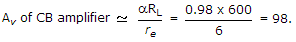37.

A bridge rectifier circuit has input of 50 Hz frequency. The load resistance is RL and filter capacitance is C. For good output wave shape, the time constant RLC should be at least equal to

 A. 10 ms B. 20 ms C. 50 ms D. 100 ms

Explanation:

Time constant RLC must be about 5 x 20 ms.

38.

Si transistor of following figure has a = 0.9 and ICE= 0, VEE = 5 V and VCC = 13 V, then RE will be if IEQ = 1 mA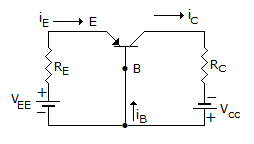A. 3 kΩ B. 4 kΩ C. 5 kΩ D. 4.3 kΩ

Explanation: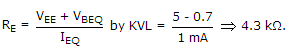39.

In class C operation of an amplifier circuit, the collector current exists for

 A. 360° of input wave B. 180° of input wave C. more than 180° of input wave D. less than 180° of input wave

Explanation:

In class C operation output is in the form of short duration pulses.

40.

The 'h' parameters of the circuit shown in the figure are hib = 25 Ω, hfb = 0.999 and hob = 10-6Ω The Voltage gain is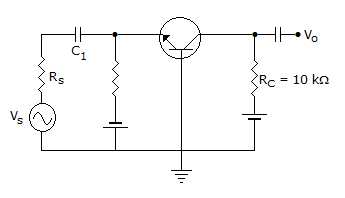A. 0.99 B. 1.98 C. 2 D. 4

Explanation: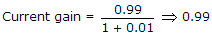Zi = 25 + 10-6 x 0.99 x 10425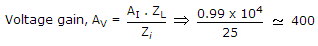.